SNAP Library 6.0, Developer Reference  2020-12-09 16:24:20 SNAP, a general purpose, high performance system for analysis and manipulation of large networks
TUNGraph::TEdgeI Class Reference

Edge iterator. Only forward iteration (operator++) is supported. More...

`#include <graph.h>`

Collaboration diagram for TUNGraph::TEdgeI: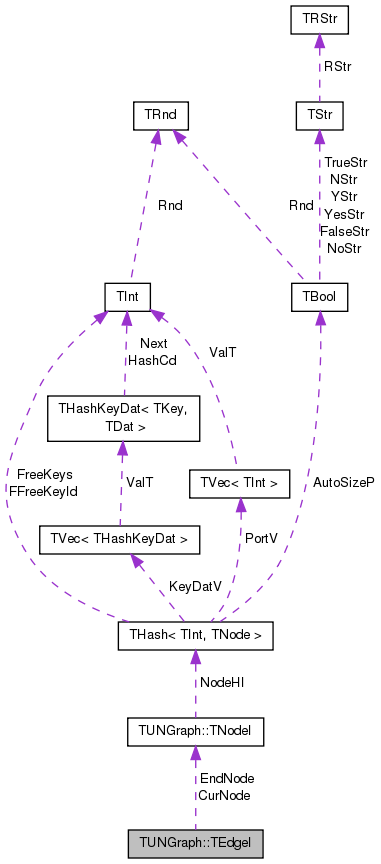[legend]

## Public Member Functions

TEdgeI ()

TEdgeI (const TNodeI &NodeI, const TNodeI &EndNodeI, const int &EdgeN=0)

TEdgeI (const TEdgeI &EdgeI)

TEdgeIoperator= (const TEdgeI &EdgeI)

TEdgeIoperator++ (int)
Increment iterator. More...

bool operator< (const TEdgeI &EdgeI) const

bool operator== (const TEdgeI &EdgeI) const

int GetId () const
Returns edge ID. Always returns -1 since only edges in multigraphs have explicit IDs. More...

int GetSrcNId () const
Returns the source of the edge. Since the graph is undirected, this is the node with a smaller ID of the edge endpoints. More...

int GetDstNId () const
Returns the destination of the edge. Since the graph is undirected, this is the node with a greater ID of the edge endpoints. More...

TNodeI CurNode

TNodeI EndNode

int CurEdge

class TUNGraph

## Detailed Description

Edge iterator. Only forward iteration (operator++) is supported.

Definition at line 121 of file graph.h.

## Constructor & Destructor Documentation

 TUNGraph::TEdgeI::TEdgeI ( )
inline

Definition at line 126 of file graph.h.

126 : CurNode(), EndNode(), CurEdge(0) { }
TNodeI EndNode
Definition: graph.h:123
TNodeI CurNode
Definition: graph.h:123
 TUNGraph::TEdgeI::TEdgeI ( const TNodeI & NodeI, const TNodeI & EndNodeI, const int & EdgeN = `0` )
inline

Definition at line 127 of file graph.h.

127 : CurNode(NodeI), EndNode(EndNodeI), CurEdge(EdgeN) { }
TNodeI EndNode
Definition: graph.h:123
TNodeI CurNode
Definition: graph.h:123
 TUNGraph::TEdgeI::TEdgeI ( const TEdgeI & EdgeI )
inline

Definition at line 128 of file graph.h.

128 : CurNode(EdgeI.CurNode), EndNode(EdgeI.EndNode), CurEdge(EdgeI.CurEdge) { }
TNodeI EndNode
Definition: graph.h:123
TNodeI CurNode
Definition: graph.h:123

## Member Function Documentation

 int TUNGraph::TEdgeI::GetDstNId ( ) const
inline

Returns the destination of the edge. Since the graph is undirected, this is the node with a greater ID of the edge endpoints.

Definition at line 139 of file graph.h.

References TUNGraph::TNodeI::GetOutNId().

139 { return CurNode.GetOutNId(CurEdge); }
TNodeI CurNode
Definition: graph.h:123
int GetOutNId(const int &NodeN) const
Returns ID of NodeN-th out-node (the node the current node points to).
Definition: graph.h:106

Here is the call graph for this function: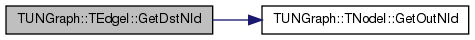Here is the caller graph for this function: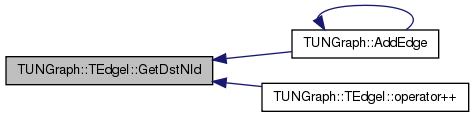int TUNGraph::TEdgeI::GetId ( ) const
inline

Returns edge ID. Always returns -1 since only edges in multigraphs have explicit IDs.

Definition at line 135 of file graph.h.

135 { return -1; }
 int TUNGraph::TEdgeI::GetSrcNId ( ) const
inline

Returns the source of the edge. Since the graph is undirected, this is the node with a smaller ID of the edge endpoints.

Definition at line 137 of file graph.h.

References TUNGraph::TNodeI::GetId().

137 { return CurNode.GetId(); }
TNodeI CurNode
Definition: graph.h:123
int GetId() const
Returns ID of the current node.
Definition: graph.h:88

Here is the call graph for this function: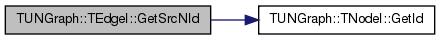Here is the caller graph for this function: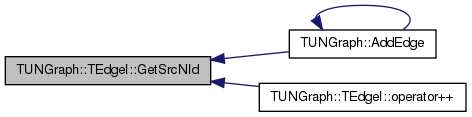TEdgeI& TUNGraph::TEdgeI::operator++ ( int )
inline

Increment iterator.

Definition at line 131 of file graph.h.

References GetDstNId(), TUNGraph::TNodeI::GetOutDeg(), and GetSrcNId().

131 { do { CurEdge++; if (CurEdge >= CurNode.GetOutDeg()) { CurEdge=0; CurNode++; while (CurNode < EndNode && CurNode.GetOutDeg()==0) { CurNode++; } } } while (CurNode < EndNode && GetSrcNId()>GetDstNId()); return *this; }
int GetDstNId() const
Returns the destination of the edge. Since the graph is undirected, this is the node with a greater I...
Definition: graph.h:139
int GetSrcNId() const
Returns the source of the edge. Since the graph is undirected, this is the node with a smaller ID of ...
Definition: graph.h:137
TNodeI EndNode
Definition: graph.h:123
int GetOutDeg() const
Returns out-degree of the current node (returns same as value GetDeg() since the graph is undirected)...
Definition: graph.h:94
TNodeI CurNode
Definition: graph.h:123

Here is the call graph for this function:bool TUNGraph::TEdgeI::operator< ( const TEdgeI & EdgeI ) const
inline

Definition at line 132 of file graph.h.

References CurEdge, and CurNode.

132 { return CurNode<EdgeI.CurNode || (CurNode==EdgeI.CurNode && CurEdge<EdgeI.CurEdge); }
TNodeI CurNode
Definition: graph.h:123
 TEdgeI& TUNGraph::TEdgeI::operator= ( const TEdgeI & EdgeI )
inline

Definition at line 129 of file graph.h.

References CurEdge, CurNode, and EndNode.

129 { if (this!=&EdgeI) { CurNode=EdgeI.CurNode; EndNode=EdgeI.EndNode; CurEdge=EdgeI.CurEdge; } return *this; }
TNodeI EndNode
Definition: graph.h:123
TNodeI CurNode
Definition: graph.h:123
 bool TUNGraph::TEdgeI::operator== ( const TEdgeI & EdgeI ) const
inline

Definition at line 133 of file graph.h.

References CurEdge, and CurNode.

133 { return CurNode == EdgeI.CurNode && CurEdge == EdgeI.CurEdge; }
TNodeI CurNode
Definition: graph.h:123

## Friends And Related Function Documentation

 friend class TUNGraph
friend

Definition at line 140 of file graph.h.

## Member Data Documentation

 int TUNGraph::TEdgeI::CurEdge
private

Definition at line 124 of file graph.h.

Referenced by operator<(), operator=(), and operator==().

 TNodeI TUNGraph::TEdgeI::CurNode
private

Definition at line 123 of file graph.h.

Referenced by operator<(), operator=(), and operator==().

 TNodeI TUNGraph::TEdgeI::EndNode
private

Definition at line 123 of file graph.h.

Referenced by operator=().

The documentation for this class was generated from the following file: